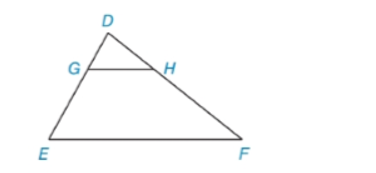Chapter 5.3, Problem 12E### Elementary Geometry for College St...

6th Edition
Daniel C. Alexander + 1 other
ISBN: 9781285195698

#### Solutions

Chapter
Section### Elementary Geometry for College St...

6th Edition
Daniel C. Alexander + 1 other
ISBN: 9781285195698
Textbook Problem
1 views

# In Exercises 9 to 12, name the method that explains why ∆ D G H ~ ∆ D E F . See the figure in the right column. ∠ D G H ≅ ∠ D E FTo determine

To name:

The method that explains why DGH~DEF

Explanation

Given:

The triangle,

Here,

DGHDEF

Approach:

Two polygons are similar if and only if two conditions are satisfied.

1. All pairs of corresponding angles are congruent.

2. All pairs of corresponding sides are proportional.

Definitions:

AA:

If the two angles of one triangle are congruent to the two angles of a another triangle, then the triangles are similar.

SAS:

If an angle of one triangle is congruent to an angle of a second triangle and the pairs of sides including the angles are proportional (in length), then the triangles are similar.

SSS:

If the three sides of one triangle are proportional (in length) to the three corresponding sides of a second triangle, then the triangles are similar.

Description:

For the given triangle,

It is given that,

DGHDEF

The given figure contains two triangles DGH and DEF

### Still sussing out bartleby?

Check out a sample textbook solution.

See a sample solution

#### The Solution to Your Study Problems

Bartleby provides explanations to thousands of textbook problems written by our experts, many with advanced degrees!

Get Started

#### Multiply: (+4)(9)

Elementary Technical Mathematics

#### Using a binomial series, the Maclaurin series for is:

Study Guide for Stewart's Multivariable Calculus, 8th

#### Sometimes, Always, or Never: If f(c) = 0, then c is a critical number.

Study Guide for Stewart's Single Variable Calculus: Early Transcendentals, 8th

#### How does a full-text database differ from other databases?

Research Methods for the Behavioral Sciences (MindTap Course List)